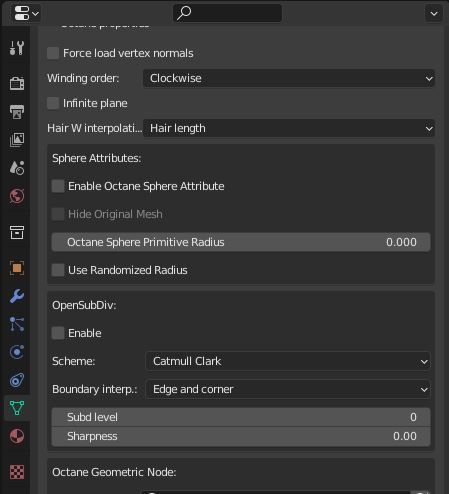# OpenSubDiv

• Enable - Activate OpenSubDiv
• Scheme - Select one of the OpenSubdiv subdivision scheme classes, which provides the methods for computing the various sets of weights used to compute new vertices resulting from subdivision.
• Catmull-Clark - A uniform refinement is applied to the Mesh faces. It subdivides the Mesh by the same amount.
• Loop - Subdivision scheme for triangular meshes, where each recursively defined subdivision surface divides into smaller ones.
• Bilinear - Subdivision scheme where the limit surface goes through the existing vertices, resulting in softened edges but no drastic changes in shape.
• Subd Level - Allows subdivision surface refinement based on Pixar’s OpenSubDiv implementation. Controls the number of times OctaneRender subdivides the original version of the Mesh.
• Sharpness - Controls the Sharpness values for the crease at and around a vertex. Crease sharpness values range from 0 (smooth) to 10 (infinitely sharp).Figure 1: OpenSubDiv parameters